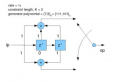# Transition table / matrix for convolutional encoder K=3 understandings (it used for RF transmitter FSK modulation)

#### JimmyCho

Joined Aug 1, 2020
109
Im trying to understand convolutional encoder K=3 (K is number of D flip flops-registers), and I almost understand it but still confused about what's called transition matrix which in other words it means " what input value (0 or 1) would produce the next state, given the current state" So if we have K=3 which means 3 shift registers, there's 2^(K-1) possibilities which means there's 4 states as Im showing down: (it's in other words state machine)And I fully understand it, and Im stuck on how do I built the correspond transition matrix for this state machine?
I've seen on the internet something weird that the transition table for convolutional encoder K=3 is:
transitionMatrix = [0 1 1 55; 0 0 1 1; 55 0 55 1; 55 0 55 1]; it was written that value 55 is value of STATE HISTORY . I didn't understand this matrix and how this matrix describe the state machine that I attached the photo above for convolutional encoder K=3